Next: Elastic or Spring Potential Up: Potential Energy Previous: Potential Energy

## Gravitational Potential Energy

The simplest conservative'' force is the force of attraction between an object and the earth when the object is in free fall. Suppose such an object falls from a height Y1 above the ground to a height Y2, as in the following figure.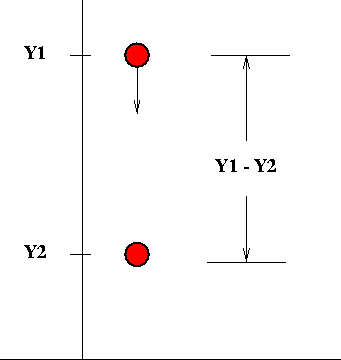The work done on this object is that due to the force of gravity, which we recall is the object's weight, multiplied by the distance covered: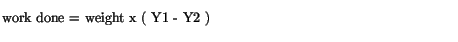One defines the gravitational potential energy of the object as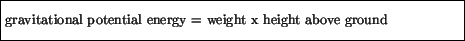for which we then haveThe total energy in this case is the sum of the kinetic and gravitational potential energy, and is conserved. However, when an object is in free fall, for example, the kinetic and gravitational potential energy are constantly changing, and thus can transform into one another.

Let us illustrate this latter point with an object falling. On its way down the object loses height and gains speed; it thus loses gravitational potential energy and gains kinetic energy. One can then say that the gravitational potential energy is being transformed to kinetic energy as the object falls. On the other hand, if an object is thrown upwards, it gains height and loses speed, thus increasing its gravitational potential energy and decreasing its kinetic energy. In this case it is the kinetic energy which is being transformed into gravitational potential energy. However, in either case, at any given point in time one finds that the sum of the kinetic and gravitational potential energy remains the same.

Using the figures for the motion of an object in free fall from the previous chapter, graphs of the various energies of this motion are as follows.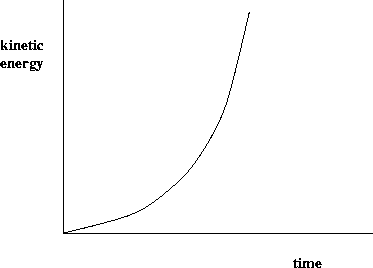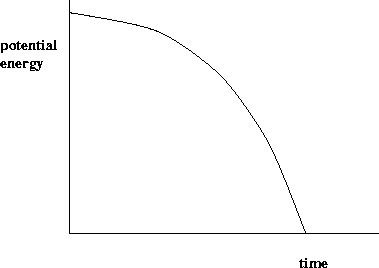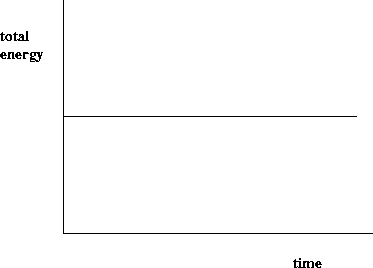Next: Elastic or Spring Potential Up: Potential Energy Previous: Potential Energy
modtech@theory.uwinnipeg.ca
1999-09-29